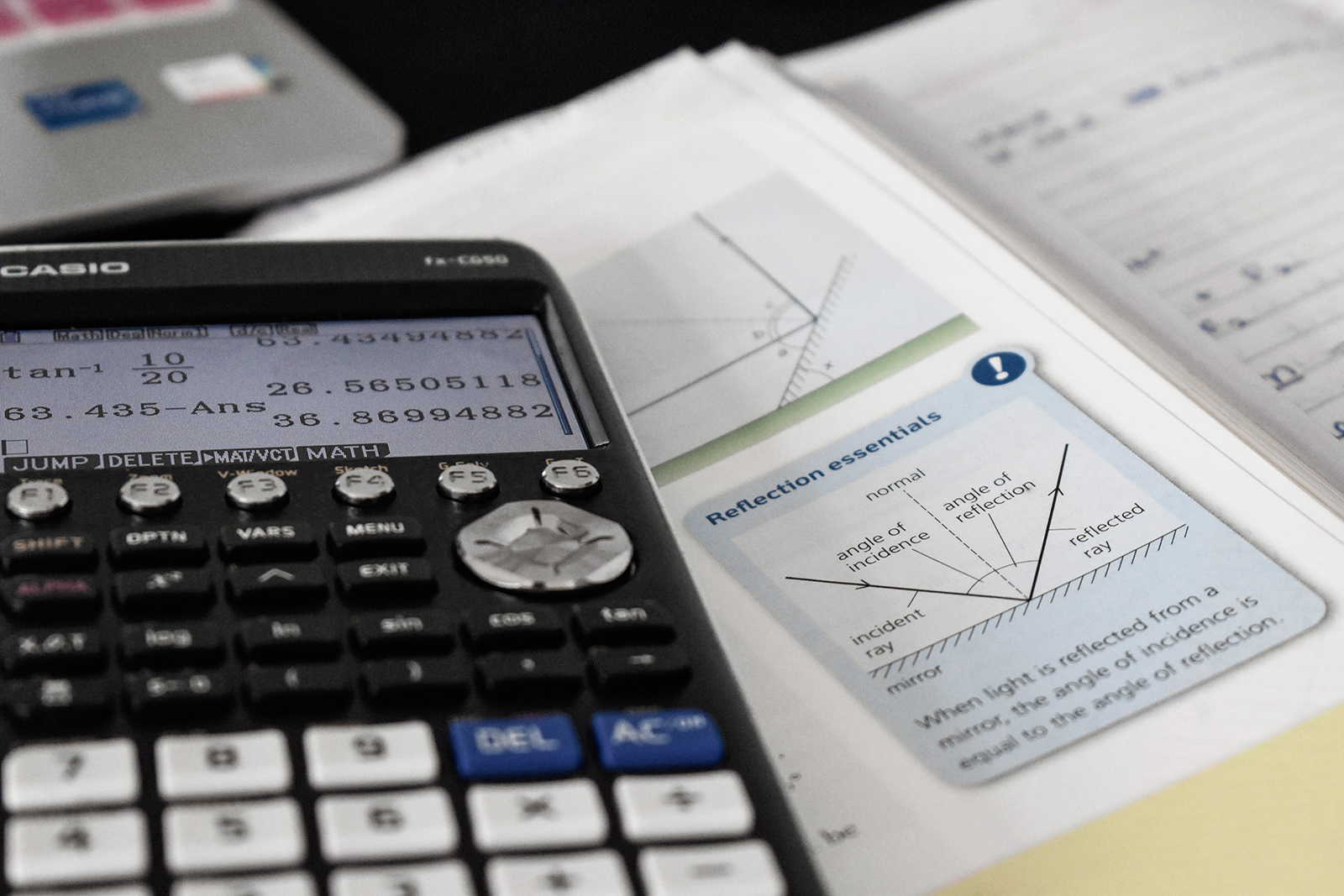##### Tutorial | Basic Trigonometry for Game Development

Basic Trigonometry for Game Development Trigonometry derives from Greek words tigonon or Sanskrit word trikona both meaning triangle and metron from Greek or matra from Sanskrit, both meaning measurement. India created the earliest known tables of values of trigonometric functions such as sine and cosine. So why do we need to know about trigonometry in... » read more